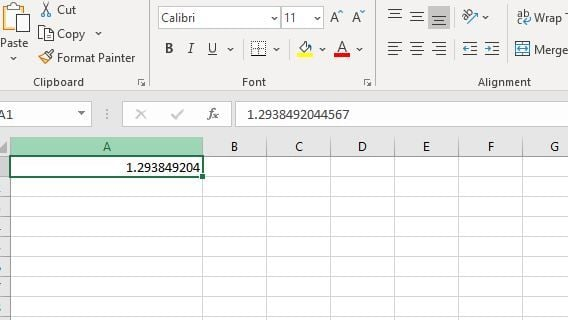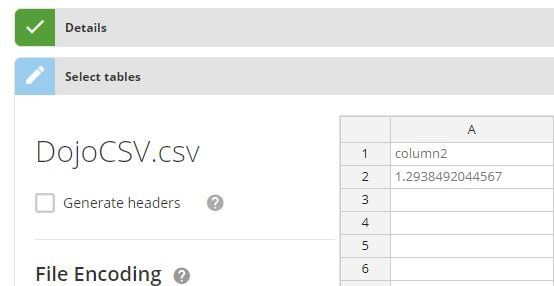Numbers fail to round up upon the usage of Excel connector

I have some values in a CSV file which are rounded up. But upon uploading the data via CSV connector the values do not show rounded up I see number goes upto 5 to 8 exact decimal places. I there way to avoid this and get the values rounded up as in the source excel file.
Thank you

• I'm trying to figure out of this is a Domo thing or an Excel thing. Maybe provide some example data.

If I open Excel and type in a long decimal place the cell shows the shortened 1.293849204 and the formula bar shows the full number I typed in 1.2938492044567. Once I save the file as a CSV and reopen it in Excel both the cell and formula bar show the shortened number. When I upload it to Domo, as I expect based on what the saved csv file contains it shows as the shortened rounded number in Domo.If I open a blank text file and paste in the same thing in a CSV format, save it as a CSV, and upload to Domo it shows the full numberRegardless of what is actually causing this you have a few options.

1: Calculated field to round the numbers when visualizing.

• You have the ROUND() method available where you provide the column, and the number of decimals you want to show. Ex. ROUND(`column`,5) to round to 5 decimal places

2: Dataflow to change the data once it's in domo. You can use a MySQL dataflow using it's ROUND() function where you do the same thing, provide it a column and the amount of decimals you want to round it to. This would create a new dataset where all the raw data is rounded as you're asking, then when you visualize you don't have to do anything additional.

• Your dataflow would be something like
• SELECT
*
,ROUND(`column`,5) as 'RoundedColumn'
FROM TABLE